# MA.912.NSO.1.1Export Print
Extend previous understanding of the Laws of Exponents to include rational exponents. Apply the Laws of Exponents to evaluate numerical expressions and generate equivalent numerical expressions involving rational exponents.

### Clarifications

Clarification 1: Instruction includes the use of technology when appropriate.

Clarification 2: Refer to the K-12 Formulas (Appendix E) for the Laws of Exponents.

Clarification 3: Instruction includes converting between expressions involving rational exponents and expressions involving radicals.

Clarification 4:Within the Mathematics for Data and Financial Literacy course, it is not the expectation to generate equivalent numerical expressions.

General Information
Subject Area: Mathematics (B.E.S.T.)
Strand: Number Sense and Operations
Status: State Board Approved

## Benchmark Instructional Guide

• Base
• Exponent
• Expression

### Vertical Alignment

Previous Benchmarks

Next Benchmarks

### Purpose and Instructional Strategies

In grade 8, students generated equivalent numerical expressions and evaluated expressions using the Laws of Exponents with integer exponents. In Algebra I, students work with rational-number exponents. In later courses, students extend the Laws of Exponents to properties of logarithms.
• Instruction includes using the terms Laws of Exponents and properties of exponents interchangeably.
• Instruction includes student discovery of the patterns and the connection to mathematical operations and the inverse relationship between powers and radicals (MTR.5.1)
• Problem types include having a fraction, integer or whole number as an exponent.
• Students should make the connection of the root being equivalent to  unit fraction exponent (MTR.4.1).
• For example, $\sqrt{8}$ = $\sqrt{2³}$ is equivalent to the equation $\sqrt{8}$ = (2³)$\frac{\text{1}}{\text{3}}$ which is equivalent to the equation $\sqrt{8}$ = 2$\frac{\text{3}}{\text{1}}$.$\frac{\text{1}}{\text{3}}$ which is equivalent to the equation $\sqrt{8}$ = 21 which is equivalent to the equation $\sqrt{8}$ = 2.

• When evaluating, students should be encouraged to approach from different entry points and discuss how they are different but equivalent strategies (MTR.2.1).

• For example, if evaluating (-27)$\frac{\text{2}}{\text{3}}$  students can either take the cube root of -27 first or raise -27 to the second power first.

### Common Misconceptions or Errors

• Students may not understand the difference between an expression and an equation.
• Students may try to perform operations on bases as well as exponents.
• Students may multiply the base by the exponent instead of understanding that the exponent is the number of times the base occurs as a factor.
• Students may not truly understand exponents that are zero or negative.

### Strategies to Support Tiered Instruction

• Teacher provides a review of the relationship between the base and the exponent by modeling an example of operations using a base and exponent.
• For example, determine the numerical value of 63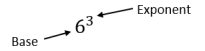• 63 which is equivalent to 6 6 6 which is equivalent to 216.
• Teacher provides exploration of the rules of exponents through patterns. A strategy for developing meaning for integer exponents by making use of patterns is shown below: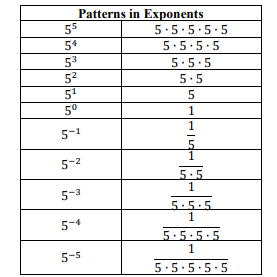• Teacher provides exploration of the rules of rational exponents through patterns. A strategy for developing meaning for rational exponents by making use of patterns is shown below: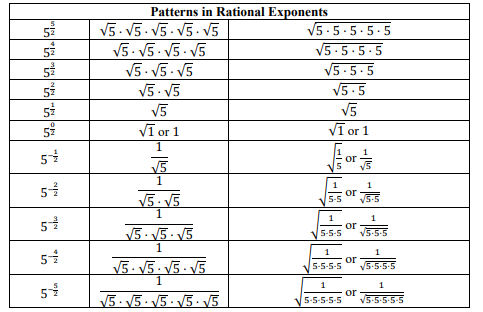• Part A. Think about when solving an equation with a radical. What is the inverse operation of a square root? Of a cube root?
• Part B. Given the expression $\sqrt{27}$, express 27 as a prime number with natural-number exponent.
• Part C. How can we use the information from Part A and B to convert $\sqrt{27}$ to exponential form?
• Part A. Evaluate 64$\frac{\text{1}}{\text{3}}$ by first writing 64 as a power of 2 and using the properties of exponents.
• Part B. Evaluate 64$\frac{\text{1}}{\text{3}}$ using a calculator.
• Part C. Explain your process in both Part A and Part B. Define powers with fractional exponents in your own words.

• Part A. Given $f$($x$) = 32$x$, evaluate $f$(0), $f$(0.2), $f$(0.4), $f$(0.8) and $f$(1) without the use of a calculator.
• Part B. Graph the function $f$ in the domain 0 ≤ $x$ ≤ 1.
• Part C. Between which two values in Part A would $f$(0.5) be? Which one would it be closer to on the graph and why?

### Instructional Items

Instructional Item 1
• Evaluate the numerical expression (64)$\frac{\text{4}}{\text{3}}$
Instructional Item 2
• Rewrite 80.5  2$\frac{\text{2}}{\text{5}}$  as a single power of 2.
Instructional Item 3
• Choose all of the expressions that are equivalent to 7$\frac{\text{5}}{\text{12}}$
• a. (49$\frac{\text{1}}{\text{3}}$)(7$\frac{\text{1}}{\text{4}}$)
• b. (7$\frac{\text{2}}{\text{3}}$)(7$\frac{\text{1}}{\text{4}}$
• c. 7(7$\frac{\text{1}}{\text{4}}$)
• d.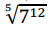• e.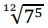Instructional Item 4

•  Evaluate the numerical expression (−$\frac{\text{729}}{\text{64}}$)$\frac{\text{2}}{\text{3}}$.

 ?
 ?
 ?

*The strategies, tasks and items included in the B1G-M are examples and should not be considered comprehensive.

## Related Courses

This benchmark is part of these courses.
1200310: Algebra 1 (Specifically in versions: 2014 - 2015, 2015 - 2022, 2022 and beyond (current))
1200320: Algebra 1 Honors (Specifically in versions: 2014 - 2015, 2015 - 2022, 2022 and beyond (current))
1200380: Algebra 1-B (Specifically in versions: 2014 - 2015, 2015 - 2022, 2022 and beyond (current))
1200400: Foundational Skills in Mathematics 9-12 (Specifically in versions: 2014 - 2015, 2015 - 2022, 2022 and beyond (current))
7912090: Access Algebra 1B (Specifically in versions: 2014 - 2015, 2015 - 2018, 2018 - 2019, 2019 - 2022, 2022 and beyond (current))
1200315: Algebra 1 for Credit Recovery (Specifically in versions: 2014 - 2015, 2015 - 2022, 2022 and beyond (current))
1200385: Algebra 1-B for Credit Recovery (Specifically in versions: 2014 - 2015, 2015 - 2022, 2022 and beyond (current))
7912075: Access Algebra 1 (Specifically in versions: 2014 - 2015, 2015 - 2018, 2018 - 2019, 2019 - 2022, 2022 and beyond (current))
1200388: Mathematics for Data and Financial Literacy Honors (Specifically in versions: 2022 and beyond (current))
1200384: Mathematics for Data and Financial Literacy (Specifically in versions: 2022 and beyond (current))
7912120: Access Mathematics for Data and Financial Literacy (Specifically in versions: 2022 - 2023, 2023 and beyond (current))
1200710: Mathematics for College Algebra (Specifically in versions: 2022 and beyond (current))

## Related Access Points

Alternate version of this benchmark for students with significant cognitive disabilities.
MA.912.NSO.1.AP.1: Evaluate numerical expressions involving rational exponents.

## Related Resources

Vetted resources educators can use to teach the concepts and skills in this benchmark.

## Formative Assessments

Roots and Exponents:

Students are asked to rewrite the square root of five in exponential form and justify their choice of exponent.

Type: Formative Assessment

Rational Exponents and Roots:

Students asked to show that two forms of an expression (exponential and radical) are equivalent.

Type: Formative Assessment

## MFAS Formative Assessments

Rational Exponents and Roots:

Students asked to show that two forms of an expression (exponential and radical) are equivalent.

Roots and Exponents:

Students are asked to rewrite the square root of five in exponential form and justify their choice of exponent.

## Student Resources

Vetted resources students can use to learn the concepts and skills in this benchmark.

## Parent Resources

Vetted resources caregivers can use to help students learn the concepts and skills in this benchmark.